§代数方程的性质

[代数基本定理每个复数域上n次代数方程

f(x)=a0xn+a1xn-1+L+an1x+an=0            (n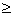1)

[多项式的导数多项式f(x)的导数为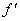(x)=na0xn1+(n1)a1xn2+L+an1

[单根与重根]

x1,x2,L,xk分别为f (x)α1,α2,L,αk(α1+α2+L+αk=n)重根，则

f (x)=a0(xx1)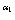(xx2)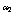L(xxk)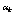[洛尔定理及其推论由微分学中的洛尔定理可知，在实系数方程f (x)=0的两个实根之间总有(x)=0的一个实根.

f(x)的一切根都是实的，则(x)的一切根也是实的.f(x)的相邻两根之间有(x)的一个根并且是一个单根.

f(x)的一切根都是实的，且其中有p个（计算重根）是正的，则(x)p个或

p1个正根.

[多项式的相关]

2° 多项式f (x)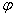(x)的根完全相同的充分必要条件是f (x)(x)只差一个不等于零的常数因子.

[整根与有理根] 任意整系数方程f (x)=0，若有一个有理根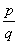（为既约分数），则pαn的约数，qα0的约数.

由此可推出：任意整系数方程的整根必为常数项的约数，若整系数方程的首项系数为1，则它的有理根必为整数.

[实根与复根，共轭实根与共轭复根]

1°  任意有理系数方程f (x)=0，若有一个根a+(a,b是有理数，是无理数)，则必有另一个根a.这时a+a称为一对共轭实根.

2° 任意实系数方程f (x)=0的复根只可能是成对的共轭复根，并且根的重数相同.从而，复根的个数是偶数.

3° 任意实系数奇数次方程f (x)=0至少有一个实根.

4° 任意实系数偶数次方程f (x)=0,a0an<0,则至少有两个实根（一个正根和一个负根）.

[根与系数的关系

f (x)=xn+a1xn1+L+an

x1+x2+L+xn==a1

x1x2+x1x3+L+xn-1xn==a2

x1x2x3+x1x2x4+L+xn-2xn-1xn==a3

LLLLLL

x1x2Lxn=(1)nan

[根的范围ξ为复系数代数方程

f (x)=a0xn+a1xn-1+L+an-1x+an=0                                        (1)

1° 若所有系数ai0 (i=0,1,L,n),,其中为实系数代数方程

F(x)=xn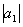xn1-L-=0

2° γ1,γ2,L,γn-1为任意正数，则τ,其中τ为下列n个数中最大的一个:+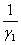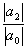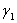+L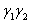L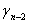+,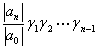max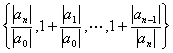(2)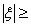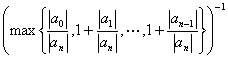(3)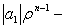L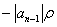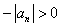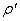>m，使得L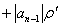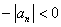3° γ为任意正数，则，其中

τ1=max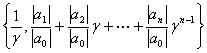4° 若所有系数都为正实数，则

min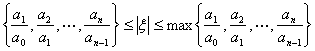5° 若方程（1）的系数满足不等式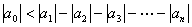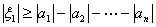[多项式的分解]

1° f (x)为实数域上的多项式，若有非常数的实系数多项式g(x)h(x),使得

f (x)=g(x)h(x)

2° 实数域上不可约多项式，除一次多项式外，只有含（共轭）复根的二次多项式.

3° 每个实系数多项式都可分解为实系数的一次因式与二次因式之积.

有理数域上的多项式的分解见第二十章，§52.

[余数定理与综合除法c为一常数，则多项式f (x)除以xc所得的余数等于f(c).

f (x)=a0xn+a1xn-1+L+an1x+an

f (x)除以xc的商式与余数其计算格式如下：

c)   a0   a1   a2   L   an-1   an

b0c  b1c  L   bn-2c   bn-1cb0   b1   b2   L   bn-1   bn

f (x)除以 x2. 列出算式

2)  1    2   3    0   5

2    8    10    201    4    5    10    15= f (2)

[多项式的泰勒公式（秦九韶法）n次多项式

f (x)=a0xn+a1xn-1+L+an-1x+an          (a00)

f (x)=b0(xc)n+b1(xc)n-1+L+bn-1(xc)+bn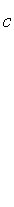ai,jc+ai,j+1=ai+1,j+1  (i=2,L,n+1; j=1,L,i1)

b0=a0bi=an+2,i+1    (i=1,2,L,n)

f (x)x=2处的泰勒展开式.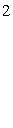f (x)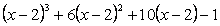[多元多项式设常数c1,c2,L,ck属于一个数域S,αi,βi,L,νi(i=1,2,L,k)是正整数或零，则称形如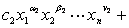L+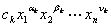每个m次多项式f(x1,x2,L,xn)都可唯一地表示成

f(x1,x2,L,xn)=为了方便，经常把一个多元多项式按某一个变数，例如x1的降幂排列如下：

a0(x2,L,xn)x1m+ a1(x2,L,xn)x1m-1+L+ am(x2,L,xn)

f1,f2,L,fk分别为m1,m2,L,mk次的多元多项式，则乘积f1f2Lfkm1+m2+L+mk.

[对称多项式如果在一个n元多项式f(x1,x2,L,xn)中，对调任一对xixj后，f(x1,x2,L,xn)不变，那末称它为x1,x2,L,xn的对称多项式.

[初等对称多项式LLLLLL           σn=x1x2Lxn

[对称多项式基本定理在数域S上，每个n元对称多项式f(x1,L,xn)都可唯一地表成x1,L,xn的初等对称多项式（系数在S中）的多项式.

[牛顿公式]

f(x)=(xx1) (xx2)L (xxn)=xnσ1xn1+L+(1)nσn

sk=x1k+x2k+L+xnk        (k=0,1,2,L)

kn,   skσ1sk-1+σ2sk-2+L+(1)k-1σk-1s1+(1)kkσk =0

k>n时，  skσ1sk-1+σ2sk-2+L+(1)nσnsk-n=0

[结式]

f(x)=a0xm+a1xm1+L+am=a0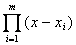(m>0)

j(x)=b0xn+b1xn1+L+bn=b0(n>0)

R(f,j)=R(f,j)=(1)mn R(j,f)

R(f,j)=a0,b0不全为零，则f(x),j(x)在复数域上有公共根的充分必要条件是它们的结式R(f,j)=0.

行列式R(f,j)f(x)j(x)的系数的一个m+n次齐次多项式，关于a0,a1,L,amn次齐次多项式，关于b0,b1,L,bnm次齐次多项式.

[傅立叶-布当判别法f(x)=0为实系数n次代数方程，a,b为二实数，适合a<bf(a)0,f(b)0,f(x)的各阶导数为

f(x),(x),L,f (n)(x){ f(b),(b),L,f (n)(b)}

*序列的变号次数定义如下：设两个相邻数都不为零，它们的符号相反，则称两数之间有一次变号，否则变号次数为零.如果遇到零时则应考虑该数后面第一个非零数是否变号.也就是说把序列中的一切零去掉再考虑变号次数.

特别，当p-q=0时，(a,b)内无实根，当pq=1时，(a,b)内只有一个实根.

[笛卡儿符号法则

f(x)=a0xn+a1xn-1+L+an=0                (a00,an0)

{a0,a1,L,an}

特别，当p=0时，无正根，当p=1时，有且仅有一个单正根.

上面两个定理没有解答这样的问题：一个给定的实系数方程是否有实根，有几个实根，并且在给定的区间(a,b)内有几个实根.斯图姆解决了这些问题.

[斯图姆判别法f(x)为区间(a,b)内的无重根的实系数多项式，a,b为二实数，适合a<b,f(a)0,f(b)0,f0(x)表示f(x)，以f1(x)表示f(x)的导数(x).f1(x)f(x)，并以f2(x)表示由这个除法所得到的余式反号后的多项式，然后用f2(x)f1(x)，并以f3(x)表示余式反号后

{f0(x),f1(x),f2(x),L,fs(x)}                                              (1)

若序列

{f0(a),f1(a),f2(a),L,fs(a)}

{f0(b),f1(b),f2(b),L,fs(b)}

应用斯图姆判别法可以查清实系数代数方程的根在实轴上的分布情况.特别，可以求出一组区间，使得每个区间内只含有方程的一个根.

关于代数方程f(z)=0的复根个数可参看第十章，§4，二的辐角原理.

[卢斯判别法假设实系数多项式

f(z)=zn+a1zn1+L+an1z+an

f0(t)=t na2tn2+a4tn4-a6tn6+L

f1(t)=a1tn1a3tn3+a5tn5L

{f0(t),f1(t),f2(t),L,fs(t)}                                          (2)

1° f(z)=0在虚轴及右半平面上没有根的充分必要条件是：斯图姆组（2）内s=n，且每个多项式的次数比前一个低一次，首项系数都是正数.

2° 若斯图姆组（2）内s=n，则组内每个多项式的次数比前一个低一次，f(z)=0在虚轴上没有根，在右半平面的根的个数等于首项系数组成的序列的变号次数.

3° f(z)=0在右半平面上没有根而在虚轴上有p个根的充分必要条件是：斯图姆组（2）内s=n-p，且每个多项式的次数比前一个低一次，首项系数都是正数，且最后的p次方程

fnp(z)=0p个实根.这些实根就是f(z)=0在虚轴上的p个根的虚部.

如果考虑f(z)=0在单位圆上和单位圆外的根数问题，只要作线性变换

z=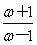[胡尔威茨判别法实系数多项式

f(z)=zn+a1zn1+L+an

f0(t)=tna2tn2+a4tn4+L

f1(t)=a1tn1a3tn3+a5tn5L